# GSEB Solutions Class 6 Maths Chapter 9 Data Handling Ex 9.1

Gujarat Board GSEB Textbook Solutions Class 6 Maths Chapter 9 Data Handling Ex 9.1 Textbook Questions and Answers.

## Gujarat Board Textbook Solutions Class 6 Maths Chapter 9 Data Handling Ex 9.1

Question 1.
In a Mathematics test, the following marks were obtained by 40 students. Arrange these marks in a table using tally marks.(a) Find how many students obtained marks equal to or more than 7.
(b) How many students obtained marks below 4?
Solution:
We have the following table: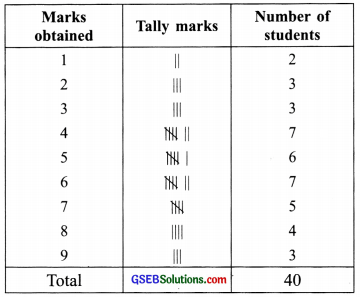(a) From the above table, we can say that 5 + 4 + 3, i.e. 12 students obtained marks equal to or more than 7.
(b) 2 + 3 + 3, i.e. 8 students obtained marks below 4.Question 2.
Following is the choice of sweets of 30 students of Class VI.
(a) Arrange the names of sweets in a table using tally marks.
(b) Which sweet is preferred by most of the students?
Solution:
(a) We have the following table:(b) From the table, we can say that Ladoo is preferred by most of the students.Question 3.
Catherine threw a dice 40 times and noted the number appearing each time as shown below: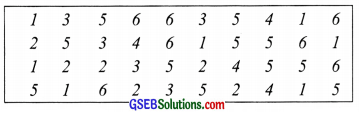Make a table and enter the data using tally marks. Find the number that appeared:
(a) The minimum number of times.
(b) The maximum number of times.
(c) Find those numbers that appear an equal number of times
Solution: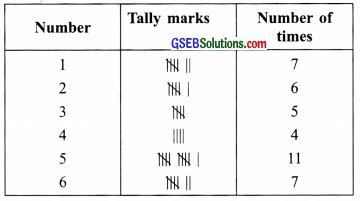From the table we find that:
(a) The number 4 appeared a minimum number of times.
(b) The number 5 appeared maximum number of times.
(c) The number 1 and 6 appear the same number of times.Question 4.
The following pictograph shows the number of tractors in five villages.Observe the pictograph and answer the following questions.
(i) Which village has the minimum number of tractors?
(ii) Which village has the maximum number of tractors?
(iii) How many are more tractors village C has as compared to village B?
(iv) What is the total number of tractors in all five villages?
Solution:
Observing the pictograph, we have
(i) Village D has the minimum number of tractors.
(ii) Village C has the maximum number of tractors.
(iii) ∵ 8 – 5 = 3
∴ Village C has 3 tractors more as compared to village B.
(iv) ∵ 6 + 5 + 8 + 3 + 6 = 28
∴ 28 tractors are there in all five villages.Question 5.
The number of girl students in each class of a co-educational middle school is depicted by the pictograph: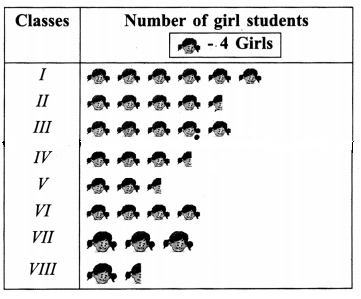Observe this pictograph and answer the following questions:
(a) Which class has the minimum number of girl students?
(b) Is the number of girls in Class VI less than the number of girls in Class V?
(c) How many girls are there in Class VII?
Solution:
From the pictograph, we find that:
(a) Class VIII has the minimum number of girl students.
(b) No, the number of girls in Class VI is not less than the number of girls in Class V.
(c) In Class VII, there are 12 girls.Question 6.
The sale of electric bulbs on different days of a week is shown below: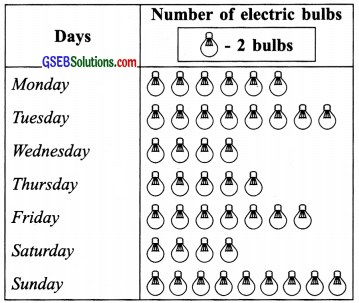Observe the pictograph and answer the following
questions:
(a) How many bulbs were sold on Friday?
(b) On which day were the maximum number of bulbs sold?
(c) On which of the day’s the same number of bulbs were sold?
(d) On which of the day’s minimum number of bulbs were sold?
(e) If one big carton can hold 9 bulbs. How many cartons were needed in the given week?
Solution:
(a) 14 bulbs were sold on Friday.
(b) the Maximum number of bulbs (18 bulbs) were sold on Sunday.
(c) On Wednesday and Saturday the same number of bulbs were sold.
(d) the Minimum number of bulbs (8 bulbs) were sold on Wednesday and Saturday.
(e) ∵ 12 + 16 + 8 + 10 + 14 + 8 + 18 = 86
∴ 86 bulbs in all were sold during the week. 10 cartons were needed in the given week.Question 7.
In a village, six fruit merchants sold the following number of fruit baskets in a particular season.Observe this pictograph and answer the following questions:
(a) Which merchant sold the maximum number of baskets?
(b) How many fruit baskets were sold by Anwar?
(c) The merchants who have sold 600 or more baskets are planning to buy a godown for the next season. Can you name them?
Solution:
Observing the pictograph, we have:
(a) Martin sold the maximum number of baskets.
(b) Anwar sold 7 × 100 i.e. 700 fruit baskets.
(c) Anwar, Martin, and Ranjit Singh are planning to buy a godown.